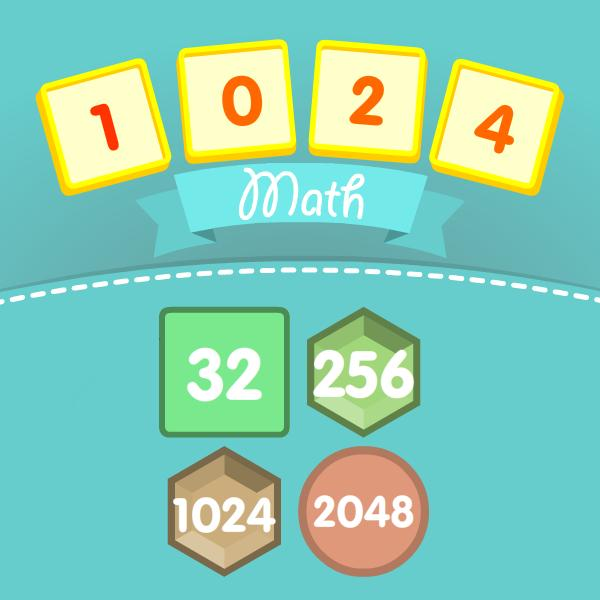# Cool Math Games

Welcome to the section "Math Games". In this section of the website, you will find mathematical games. On PlayCoolMath.com, learning math is limited to three tasks - these are lessons, tests, and games.

Games help you learn math, practice math skills. Learning math in a game form is more effective than simply listening to a boring teacher. Games are not a substitute for lessons or tests, games supplement them and allow a child or an adult to associate mathematics with the game - it positively affects the progress of training. If you think that math is difficult and boring, then you need to play our mathematical games and you will change your mind.

We create exclusive mathematical games that will allow us to consolidate the acquired material. To go to the next level in the game you need to solve the mathematical expression. If you find a correct solution, you can play more. If you make a mistake, you will be solving the task until you finally solve it. In the game settings, you can choose what mathematical operations will be, their number, and range of numbers.

## Math Mahjong Relax

Welcome to online Math Mahjong Relax. Math Mahjong Relax is a traditional solitaire game where you have to take apart the construction made of dice. The game helps to improve your attention, speed and math skills. Dice differ...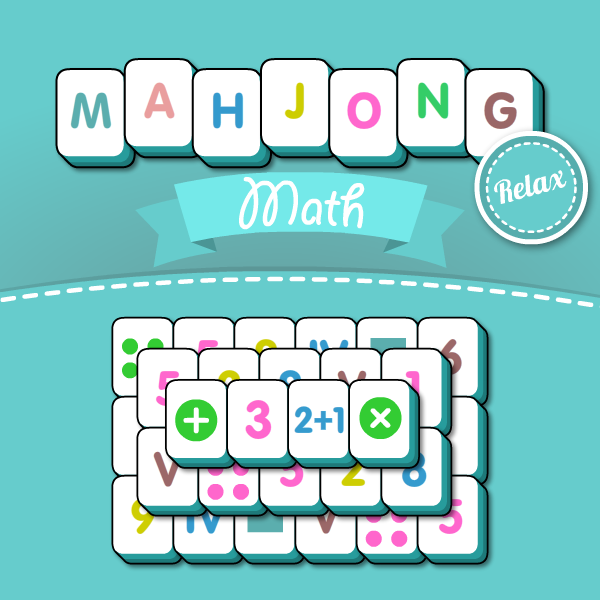## Math Number Challenge

In this game, you need be finding figures for speed. It looks quite easy for the first time. However, at every level new tasks appear complicating the search for numbers. In the game, you will learn figures in the decimal system. You will find out how ...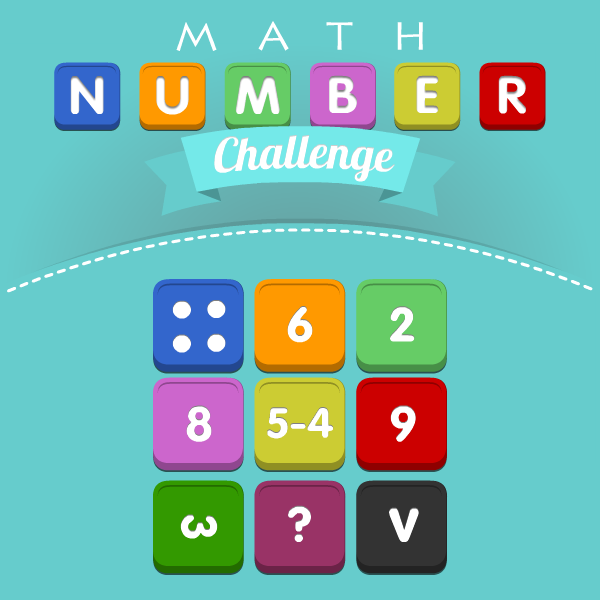## Math Pixel Puzzle

Math pixel puzzle is a very unusual game. The rules are very simple. You will see an image with pixels of various colors. After three seconds, the pixels fly apart in 3D space. Your task is to rotate the space so that ...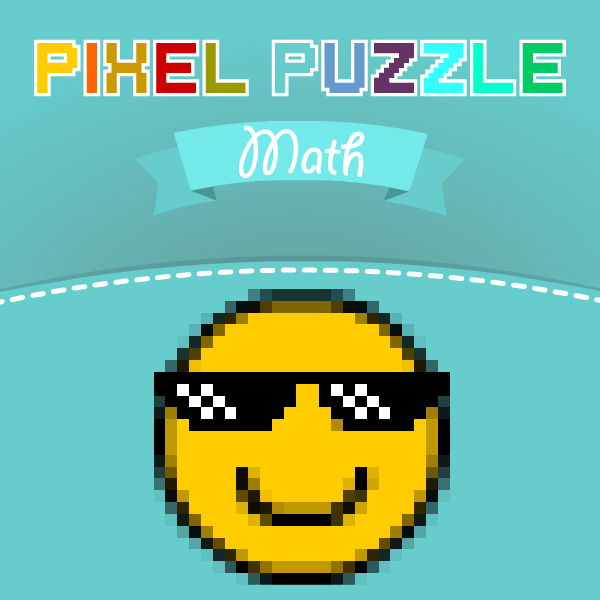## Math Tower of Hanoi

The Tower of Hanoi is a puzzle game invented in 19th century by the French mathematician Eduard Lucas. This guy got inspired by the legend about priests in Hindi castle. Those priests got 3 bars, one ...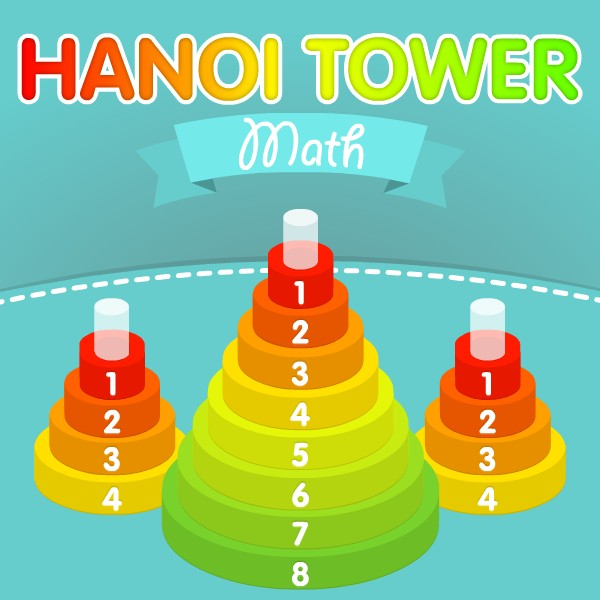## 1024 Math

1024 Math reminds a game of 2048 from Gabriele Cirulli. There are 18 levels in this game. You need to complete all the goals when you get at each level. You can move all the blocks up, down, left or right. You can...